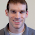## Friday, October 21, 2011

### Phylogenetic signal with measurement error

I just posted a new version of the function phylosig() that computes phylogenetic signal using Blomberg's K (Blomberg et al. 2003) and Pagel's λ methods.

The main improvement of this version is that I have added estimation of K with known measurement error, following Ives et al. (2007). This was in response to a user's email request.

This was not too difficult. Basically, without measurement error the values for the tip states on the tree under BM are distributed as a multivariate normal with variance-covariances given by σ2C, where C is an n×n matrix (for n species) containing the height above the root node of the common ancestor of each i,jth species pair on the tree. (For diagonal elements, these are just the heights of each tip node.)

With measurement error, the picture complicates slightly. Now, say the VCV matrix for the measurement error is given by M, then the multivariate distribution of the tip values is given by σ2C+M. The only problem with this is that although we have an analytic solution for σ2 conditioned on no measurement error, we do not for known M. Instead, we have to find σ2 by other means - say, by maximizing the likelihood. This is what I have done in the latest version of phylosig() (v0.4), available here.

> require(phytools)
> source("phylosig.R")

Now, just for fun, let's simulate data with measurement error.

> tree<-drop.tip(birthdeath.tree(b=1,d=0,taxa.stop=301),"301")
> x<-fastBM(tree) # simulate data
> se<-rchisq(n=300,df=1) # simulate SEs
> names(se)<-names(x)
> e<-rnorm(n=300,sd=se)
> xe<-x+e

We have data generated without error (in x) and with known error (in xe). First, let's compute phylogenetic signal for x:

> phylosig(tree,x)
 1.013599

This is more or less what we expect. Now let's try xe, but with measurement error ignored:

> phylosig(tree,xe)
 0.2640041

Finally, we can try our new function incorporating measurement error:

> phylosig(tree,xe,se=se)
\$K
 1.121445

\$sig2
 0.9772118

\$logL
 -543.0133

Very cool! Note that the logL reported here is the log-likelihood for the value of sig2 conditioned on the known measurement error we've provided.

Of course, this is not magic - so the more measurement error we have, the less power we will have to measure phylogenetic signal. That said, incorporating measurement error has the nice property of making our estimates of signal unbiased (whereas they are biased downwards when signal is ignored).

1.2.